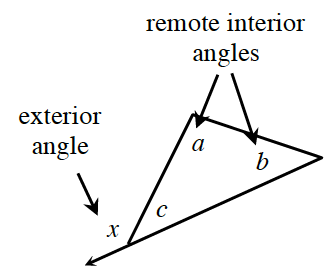Home > GC > Chapter 8 > Lesson 8.1.1 > Problem8-7

8-7.

After solving for $x$ in each of the diagrams in problem 8‑6, Jerome thinks he sees a pattern. He notices that the measure of an exterior angle of a triangle is related to two of the angles of a triangle. Homework Help ✎

1. Do you see a pattern? To help find a pattern, study the results of problem 8‑6.

Pick examples of angles that could realistically be placed in for $a$ and $b$ (remote interior angles) in the triangle above.
For example, $35º$ and $75º$ calculate the value for $x$ (the exterior angle) using the work you did in problem 8-6.
Is there a connection between $a$ and $b$ (remote exterior angles) and $x$ (exterior angle)?

If you picked other angles for $a$ and $b$ (remote interior angles) would your conjecture still work?

1. In the example at right, angles $a$ and $b$ are called remote interior angles of the given exterior angle because they are not adjacent to the exterior angle. Write a conjecture about the relationships between the remote interior and exterior angles of a triangle.

The measure of the exterior angle is equal to the sum of the remote interior angles.
$m\angle a + m\angle b = m\angle x$.1. Prove that the conjecture you wrote for part (b) is true for all triangles. Your proof can be written in any form, as long as it is convincing and provides reasons for all statements.